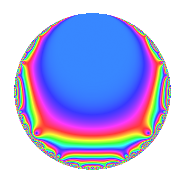# Properties

 Label 4014.2.a.kLevel 4014 Weight 2 Character orbit 4014.a Self dual Yes Analytic conductor 32.052 Analytic rank 0 Dimension 2 CM No Inner twists 1

# Related objects

## Newspace parameters

 Level: $$N$$ = $$4014 = 2 \cdot 3^{2} \cdot 223$$ Weight: $$k$$ = $$2$$ Character orbit: $$[\chi]$$ = 4014.a (trivial)

## Newform invariants

 Self dual: Yes Analytic conductor: $$32.0519513713$$ Analytic rank: $$0$$ Dimension: $$2$$ Coefficient field: $$\Q(\sqrt{5})$$ Coefficient ring: $$\Z[a_1, \ldots, a_{11}]$$ Coefficient ring index: $$1$$ Fricke sign: $$-1$$ Sato-Tate group: $\mathrm{SU}(2)$

## $q$-expansion

Coefficients of the $$q$$-expansion are expressed in terms of $$\beta = \frac{1}{2}(1 + \sqrt{5})$$. We also show the integral $$q$$-expansion of the trace form.

 $$f(q)$$ $$=$$ $$q + q^{2} + q^{4} -2 \beta q^{5} + q^{8} +O(q^{10})$$ $$q + q^{2} + q^{4} -2 \beta q^{5} + q^{8} -2 \beta q^{10} + ( 3 - 3 \beta ) q^{11} -3 \beta q^{13} + q^{16} -4 \beta q^{17} + ( 2 + \beta ) q^{19} -2 \beta q^{20} + ( 3 - 3 \beta ) q^{22} -2 q^{23} + ( -1 + 4 \beta ) q^{25} -3 \beta q^{26} + ( -1 + 5 \beta ) q^{29} + 6 q^{31} + q^{32} -4 \beta q^{34} + 4 \beta q^{37} + ( 2 + \beta ) q^{38} -2 \beta q^{40} + 10 q^{41} + ( 7 + \beta ) q^{43} + ( 3 - 3 \beta ) q^{44} -2 q^{46} + ( -5 + \beta ) q^{47} -7 q^{49} + ( -1 + 4 \beta ) q^{50} -3 \beta q^{52} + 5 \beta q^{53} + 6 q^{55} + ( -1 + 5 \beta ) q^{58} + ( -2 - 3 \beta ) q^{59} + ( 7 - 11 \beta ) q^{61} + 6 q^{62} + q^{64} + ( 6 + 6 \beta ) q^{65} + ( 2 + 8 \beta ) q^{67} -4 \beta q^{68} + ( -8 + 12 \beta ) q^{71} + ( 4 + 5 \beta ) q^{73} + 4 \beta q^{74} + ( 2 + \beta ) q^{76} + ( -3 - \beta ) q^{79} -2 \beta q^{80} + 10 q^{82} -12 q^{83} + ( 8 + 8 \beta ) q^{85} + ( 7 + \beta ) q^{86} + ( 3 - 3 \beta ) q^{88} + ( 6 - 2 \beta ) q^{89} -2 q^{92} + ( -5 + \beta ) q^{94} + ( -2 - 6 \beta ) q^{95} + ( 10 + 4 \beta ) q^{97} -7 q^{98} +O(q^{100})$$ $$\operatorname{Tr}(f)(q)$$ $$=$$ $$2q + 2q^{2} + 2q^{4} - 2q^{5} + 2q^{8} + O(q^{10})$$ $$2q + 2q^{2} + 2q^{4} - 2q^{5} + 2q^{8} - 2q^{10} + 3q^{11} - 3q^{13} + 2q^{16} - 4q^{17} + 5q^{19} - 2q^{20} + 3q^{22} - 4q^{23} + 2q^{25} - 3q^{26} + 3q^{29} + 12q^{31} + 2q^{32} - 4q^{34} + 4q^{37} + 5q^{38} - 2q^{40} + 20q^{41} + 15q^{43} + 3q^{44} - 4q^{46} - 9q^{47} - 14q^{49} + 2q^{50} - 3q^{52} + 5q^{53} + 12q^{55} + 3q^{58} - 7q^{59} + 3q^{61} + 12q^{62} + 2q^{64} + 18q^{65} + 12q^{67} - 4q^{68} - 4q^{71} + 13q^{73} + 4q^{74} + 5q^{76} - 7q^{79} - 2q^{80} + 20q^{82} - 24q^{83} + 24q^{85} + 15q^{86} + 3q^{88} + 10q^{89} - 4q^{92} - 9q^{94} - 10q^{95} + 24q^{97} - 14q^{98} + O(q^{100})$$

## Embeddings

For each embedding $$\iota_m$$ of the coefficient field, the values $$\iota_m(a_n)$$ are shown below.

For more information on an embedded modular form you can click on its label.

Label $$\iota_m(\nu)$$ $$a_{2}$$ $$a_{3}$$ $$a_{4}$$ $$a_{5}$$ $$a_{6}$$ $$a_{7}$$ $$a_{8}$$ $$a_{9}$$ $$a_{10}$$
1.1
 1.61803 −0.618034
1.00000 0 1.00000 −3.23607 0 0 1.00000 0 −3.23607
1.2 1.00000 0 1.00000 1.23607 0 0 1.00000 0 1.23607
 $$n$$: e.g. 2-40 or 990-1000 Significant digits: Format: Complex embeddings Normalized embeddings Satake parameters Satake angles

## Inner twists

This newform does not admit any (nontrivial) inner twists.

## Atkin-Lehner signs

$$p$$ Sign
$$2$$ $$-1$$
$$3$$ $$-1$$
$$223$$ $$-1$$

## Hecke kernels

This newform can be constructed as the intersection of the kernels of the following linear operators acting on $$S_{2}^{\mathrm{new}}(\Gamma_0(4014))$$:

 $$T_{5}^{2} + 2 T_{5} - 4$$ $$T_{7}$$ $$T_{11}^{2} - 3 T_{11} - 9$$